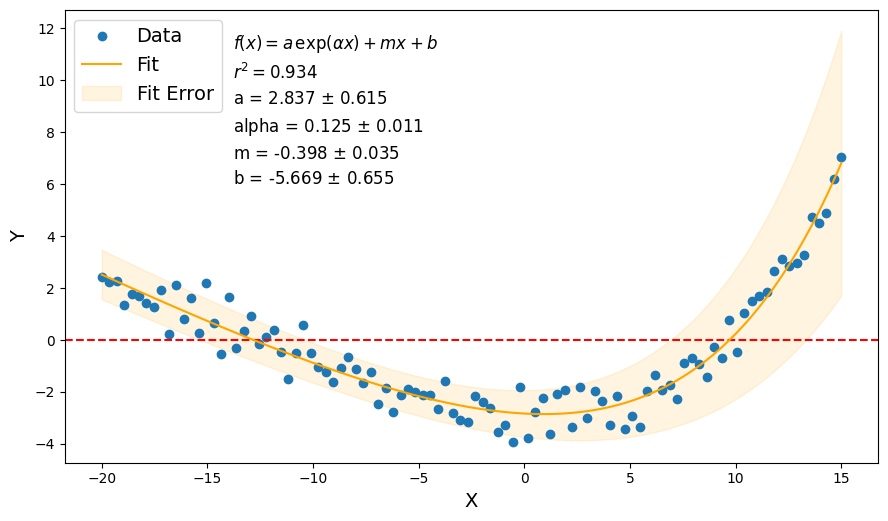# plasmapy.analysis.fit_functions

FitFunction classes designed to assist in curve fitting of swept Langmuir traces.

## Classes

 AbstractFitFunction([params, param_errors]) Abstract class for defining fit functions $$f(x)$$ and the tools for fitting the function to a set of data. Exponential([params, param_errors]) A sub-class of AbstractFitFunction to represent an exponential with an offset. ExponentialPlusLinear([params, param_errors]) A sub-class of AbstractFitFunction to represent an exponential with an linear offset. ExponentialPlusOffset([params, param_errors]) A sub-class of AbstractFitFunction to represent an exponential with a constant offset. Linear([params, param_errors]) A sub-class of AbstractFitFunction to represent a linear function.## Example Notebook